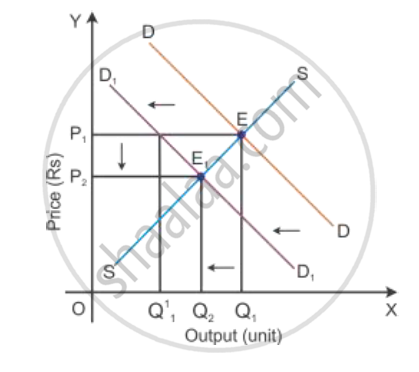# Market for a Product is in Equilibrium. Demand for the Product "Decreases." Explain the Chain of Effects of this Change Till the Market Again Reaches Equilibrium. Use Diagram - Economics

A market for a product is in equilibrium. Demand for the product "decreases." Explain the chain of effects of this change till the market again reaches equilibrium. Use diagram

A market of a commodity is in equilibrium. Demand for the commodity 'decreases'. Explain the chain of effects of this change till the market again reaches equilibrium. Use Diagram.

#### Solution

Consider DD1 to be the initial demand curve and SS to be the supply curve of the market. Market equilibrium is achieved at Point E, where the demand and supply curves intersect each other. Therefore, the equilibrium price is OP1, and the equilibrium quantity demanded is OQ1.

If there is fall in the demand, the demand curve will shift towards the left to DD1 and the supply curve SS will remain the same. This implies that at the initial price OP1, there is an excess supply of OQ1 to OQ11 units of output. Thereby the competition among producers will increase and they tend to reduce the price of the output to the OP2 level. Now, the new market equilibrium will be at Point E1, where the new demand curve DD1 intersects the supply curve SS.

This clearly states that if the demand curve shifts towards the left, the price of the good tends to decrease with the decrease in the demand for a good. Thus, the direction of change in equilibrium price and the quantity are the same whenever there is a shift in the demand curve.Concept: Market Equilibrium
Is there an error in this question or solution?